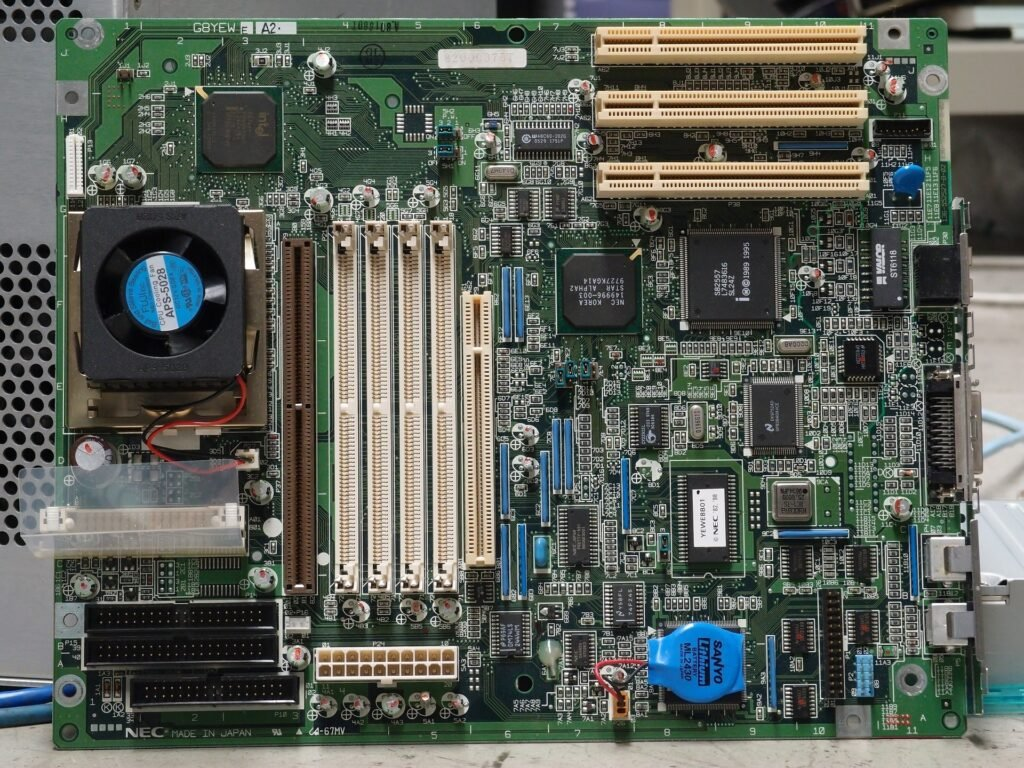# Topic 4 DiodesSilicon Diodes and Zener Diodes

Diodes are one-way electrical valves that allow current to flow in one direction only.

Click HERE to find out how diodes are made.

• In circuit A the diode is forward-biased so the current flows and the motor will turn.
• In circuit B the diode is reverse-biased, the current will not flow and the motor will not turn.
• Diodes have a low resistance when they are forward biased, and a very high resistance when they are reverse-biased.
• This means that current can flow one way only.

Now try Question 1.

The diagram below shows the four main kinds of diode:

Diodes are made from semi-conductor materials like silicon and germanium.

##### Voltage Characteristic of the Diode

The circuit above can be used to investigate the Voltage current characteristic of a diode.  The voltage and current can be recorded for the forward biased diode.  We can turn the diode round for the reverse biased diode.

A forward biased diode starts to conduct at the junction voltage (for silicon, about 0.6 V).  A small increase in the forward voltage leads to a large increase in forward current.

If we reverse the voltage, we get a tiny leakage current of no more than a few microamps.  At a certain voltage, anything from 10 V to 2000 V, depending on the doping, the insulation of the barrier layer breaks down suddenly and a sudden increase in current occurs.  The breakdown voltage is the voltage at which this happens.  In many diodes, this would result in burn-out.

Germanium diodes switch on at 2.2 V and the breakdown voltage is about 100 V.

Now try Question 2

##### Zener Diode

The zener diode is designed to be used in a reverse biased configuration.  Its behaviour is very like an ordinary diode, but a typical reverse-biased breakdown voltage is  –5.6 V, and there is a very rapid rise in current.

In its reverse biased configuration, it will hold the output voltage at a constant 5.6 V.  It can be described as a voltage clamp.  If the voltage is above 5.6 V, a current will flow through the diode, which results in the voltage being held at 5.6 V.  They are used as voltage regulators.

The graph shows how diode limits the voltage, which remains steady at 5.6 volts while a small current is taken.  The zener diode can only take a limited current.

The zener diode shown in this circuit has a maximum power rating of 400 mW, and has a reference voltage of 5.6 V .

Now try Question 3

Data Sheets

We can refer to data sheets that are found in retailers’ catalogues.   As well as price, we might get data on:

• Current that the component can carry
• Temperature that the component can tolerate.
• Case style.
• Working voltages.

Here is some data for some high power diodes from a catalogue:

 Diode Break down Voltage (V) Minimum Current (A) Maximum Current (A) Maximum Temperature (oC) Case style Price (£) SKN2.5 04 400 2.5 180 450 E5 5.63 SKN2.5 12 1200 2.5 180 450 E5 8.20 16FR 40 400 16 350 140 DO4 2.06 85HFR80 800 85 1700 125 DO5 8.85 SW15PHR400 1500 400 7500 125 DO9 21.82
##### Light Emitting Diode

The light emitting diode (LED) has replaced indicator lamps in many devices.

The forward voltage is about 2.2 V.

It is very easy to ruin LED’s with currents in excess of 30 mA.  To prevent this we put a current limiting resistor in series with the LED.

The voltage current characteristic of the LED has a similar shape to the graph of the ordinary diode.  The LED can only tolerate a small reverse voltage.  A reverse voltage of 20 V will destroy an LED.